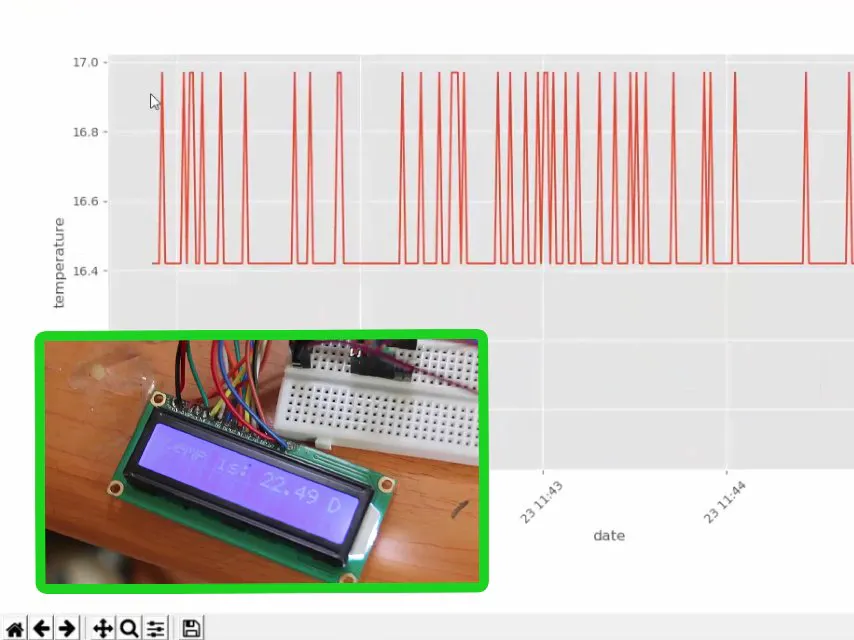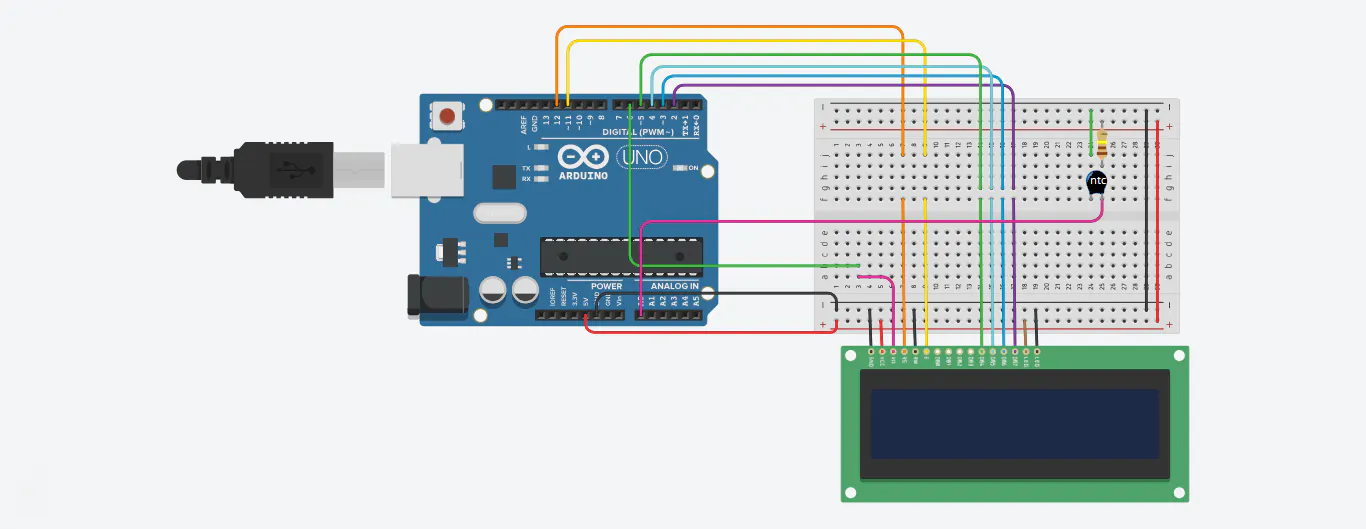Published © GPL3+

# Arduino & Python3 Temperature Data Visualizer

This is an Arduino based project using an NTC thermistor to collect temperature data and Python 3 to save and visualize it.

IntermediateFull instructions provided3,985## Things used in this project

### Hardware componentsArduino UNO & Genuino UNO
×1LED (generic)
×1
 resistor 110 ohm
×1
 ntc thermistor
×1

### Software apps and online servicesArduino IDE
 python 3 IDEL

### Hand tools and fabrication machines

 computer

Read more

## Schematics

### temperature serial## Code

### arduino_serial_temperature.ino

Arduino
this is the arduino code
``` /*

The circuit:

* LCD RS pin to digital pin 12
* LCD Enable pin to digital pin 11
* LCD D4 pin to digital pin 5
* LCD D5 pin to digital pin 4
* LCD D6 pin to digital pin 3
* LCD D7 pin to digital pin 2
* LCD R/W pin to ground
* LCD VSS pin to ground
* LCD VCC pin to 5V
* LCD K pin to ground
* LCD A pin to 5V
* LCD V0 pin to digital pin 6
* LED to pin 13

put a 110 ohm resitance in series with the thermistor, the side of 110 resitance to 5V
the side of the thermistor to GND and the point where the thermistor and the resitence meet
to analog input A0

*/

#include <LiquidCrystal.h>

LiquidCrystal lcd(12,11,5,4,3,2);//configure the lcd

#define a A0 // analog input to read the voltage of the thermistor
double b;// an intermediate variable
double Vt;
double Rt;
int R=110;
int B=2800;
int R0=5;
int T0=25;
double T;
void setup() {
analogWrite(6,120);// configure the contrast of the lcd
lcd.begin(16,2);
Serial.begin(115200);// using serial to send data to the computer
}

void loop() {
b=analogRead(a);// get the value in the A0
Vt= (b/1024)*5;// convert it to voltage
Rt=(R*Vt)/(5-Vt);// measure the resistance of the thermistor
T=1/((log(Rt/R0)/B)+(1/(T0+273.15))); // get the temperature in kelvin
Serial.println(T-273.15);// send the temperature in using serial

// desplay the temperature in the lcd
lcd.clear();
lcd.print("temp is: ");
lcd.print(T-273.15);
lcd.print(" D");

// trigger the security alarm if the temperature is higher than 60 degree
if ((T-273.15)>60){
digitalWrite(13,HIGH);
delay(500);
digitalWrite(13,LOW);
delay(500);
}
else{
delay(1000);
}
// in the both cases there will be a delay of 1s between sending the temperature to the computer and display in in the lcd
}
```

### live plot.py

Python
this is the python script for plotting the data you have to install mathplotlib library
```import matplotlib.pyplot as plt
import matplotlib.animation as animation
from matplotlib import style
import datetime as dta
'''configuring the plot'''
style.use('ggplot')
fig = plt.figure()
ax1 = fig.add_subplot(1,1,1)

def animate(i):
dateconv = dta.datetime.fromtimestamp
'''define the function that gonna convert the unix time to date'''
graph_data = open('example.txt','r').read()
'''read the data from the txt file'''
lines = graph_data.split('\n')
''' split the lines as a list'''
xs = []
''' x axis the tempurature'''
ys = []
''' y axis the date'''
for line in lines:
''' read the lines and append the data to ys ans xs'''
if len(line) > 1:
'''get only the non-empty line'''
x, y = line.split(',')
x=float(x)
y=float(y)
xs.append(x)
y = dateconv(y)
''' converte the unix time'''
ys.append(y)
'''plot the data'''
ax1.clear()
ax1.plot(ys, xs,label='temperature')
for label in ax1.xaxis.get_ticklabels():
'''set the rotation of the date into 45 degree in order to be readable'''
label.set_rotation(45)
plt.xlabel('date')
plt.ylabel('temperature')
plt.legend()
ani = animation.FuncAnimation(fig, animate, interval=1000)
'''animate the plot and refresh evry 1 second'''
plt.subplots_adjust(left=0.09, bottom=0.23, right=0.93, top=0.90, wspace=0.2, hspace=0)
'''just configurations'''
plt.show()
```

### serial colector.py

Python
this is the python script to save the data to a txt file ,you have to install serial library
```from serial import Serial
import time
'''set the serial to connect to the arduino (you should change the port)'''
ser =Serial(
port='COM8',
baudrate = 115200,
timeout=None)
def adddata(data):
'''a function to add the data to the text file'''
date=time.time()
h=str(data)+','+str(date)+'\n'
fh = open('example.txt', 'a')
fh.write(h)
fh.close
while 1:
''' infinit loop'''
while(ser.inWaiting()==0):
'''wait for the data from serial'''
pass
a=float(ser.readline().decode('utf-8'))
'''read and decode the data'''
adddata(a)
'''add the data to the txt file'''
```

## Credits

### reyadeetopee

0 projects • 6 followers